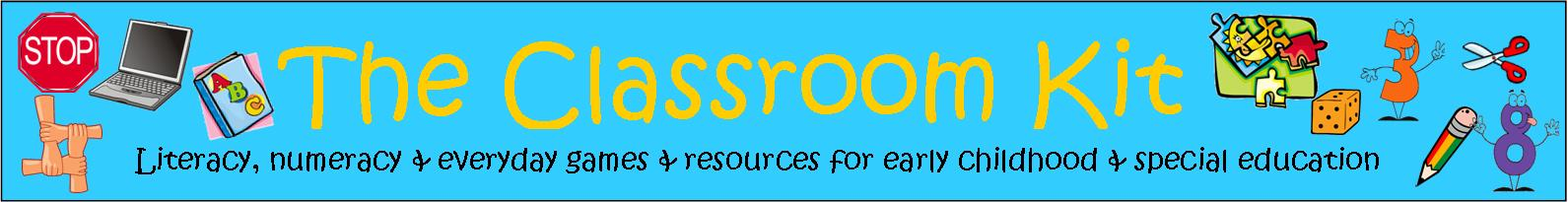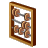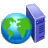## Number Worksheets and Activity Cards: Recognising Numerals and CountingNumber worksheets compliment the hands-on activities that are offered in early childhood classes. Young learners need constant opportunities to manipulate the numerals from 0 to 9, and to practise counting small groups of objects with growing accuracy. Worksheets provide teachers with hard copies of students' efforts, and are useful for assessment and reporting purposes, as evidence of understandings. It is important to include many opportunities for practising these skills within the learning program, and to build in real purposes for counting.

Activity cards support opportunities for students to count larger groups (from 11 to 20, from 20 to 50, and from 20 to 100). Teachers need to ensure that all materials necessary are available when using these cards. Once students are familiar with the procedure of each set of cards, they can work independently within small groups. This allows for different groups of students to work at their own levels of understanding within the class.

## Emergent Worksheets:

Counting Groups to 10: Count each group and write the total in each box.

### Adding small groups (up to 10 objects):

Count each group and add them together. Write the sum with the total.

### Developing the concepts of More and Less with groups from 2 to 10:

Adding 1, 2, and 3 more (with pictures) and introduction of simple algorithm (3+2=5)

What is 1,2, and 3 less (than objects shown) and introduction of simple algorithm (5-2=3)

## Perceptual Worksheets:

### Counting groups of objects from 11 to 20:

These worksheets include:

*seeing 10 as a counting unit, with 10 objects grouped together to make counting easier and more efficient

*reading and writing numbers from 11-20

### The Concept of 'Adding More' With Numbers from 11 to 20:

These worksheets include:

*adding 1, 2 or 3 more to each group

*introducing 'counting on' as a strategy to find 'more'

*writing the algorithm to represent the calculation (15+1=16)

*supporting young learners to develop 'counting on' by providing the objects to count in order to check calculations

### The Concept of 'Finding Less' With Numbers from 11 to 20:

These worksheets include:

*taking 1, 2 or 3 from each group

*introducing 'counting back' as a strategy to find 'less'

*writing the algorithm to represent the calculation (15-1=14)

*supporting young learners to develop 'counting back' by providing the objects to count in order to check calculations

Weekly Worksheets to Develop Mental Maths Skills at the Perceptual Level:

## Figurative Worksheets:

### Counting groups from 10-99

These worksheets include:

*pictorial representations of 2 digit numbers

*groups set out in lines of 10 (or arrays), to make counting easier and to encourage the strategy of counting by 10s when working with 2-digit numbers

*the numerals: 10 20 30 40 50 60 70 80 90 100 at the bottom of each page to support learners at this stage

 Groups of 3 Free resource

Weekly Worksheets to Promote Mental Maths Skills at the Figurative Level:

## Counting On Worksheets:

These worksheets include:

*pictorial representations of 2 digit numbers

*groups set out in lines of 10 (or arrays), to make counting easier and to encourage the strategy of counting by 10s when working with 2-digit numbers

*an introduction to the algorithm for adding two 2-digit numbers vertically (supported by pictorial representation of each numeral)

### Subtracting groups of 10

These worksheets include:

*pictorial representations of 2 digit numbers

*groups set out in lines of 10 (or arrays), to make counting easier and to encourage the strategy of counting by 10s when working with 2-digit numbers

*an introduction to the algorithm for subtracting two 2-digit numbers vertically (supported by pictorial representation of each numeral)

## Facile Worksheets: